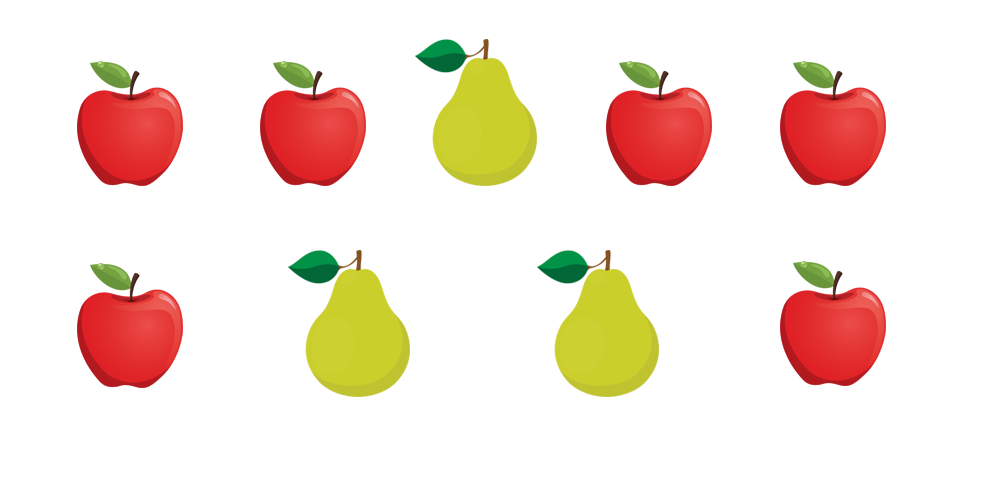Math 1
Math 2
Math 3
Math 4
Math 5
Math 6
200
How can you write nineteen and eighty-two hundredths
What is 19.82
200

What is the ratio of apples to total fruit?6:9

200

How would you say this number 381.205?

three hundred eighty-one and two hundred five thousandths

200

Solve for X:

2/3 = 8/x

X = 12

200

Solve:

5/4 + 2/8

1 1/2

200

What is (7/12) / (1/2)

Symplify as a mixed number

1 (1/6)

400

3.24 + 2.7 =

5.94
400

Write a fraction equivalent to

8/15 with a denominator of 75

40/75

400

Subtract: 80 - 39.6

What is 40.4

400

What is 215 divided by 5?

43

400

Solve:

83.781 - 15.425

68.356

400

Solve for X

X + 2.4 = 7.1

4.7

600

.056 x 1.5

.084

600

10/4

2.5

600

10 x 3.67

What is 36.7?

600
What is 8.01
600

The ratio of basketball players to soccer players is 3:5.  If there are 30 basketball players in the school, how many soccer players are there?

50

600

3/5 divided by 4

3/20

800

Write 14 3/9 as an improper fraction

129/9

800

173.45 + 2.38

175.83

800

Which value is greater? .078 or .7

What is .7

800

Simplify 9/54

1/6

800

4/5 divided by 1/3

2 2/5
800

How can you write one hundred seventeen and three tenths?

117.3

1000

Write 69/8 as a mixed number

8 5/8

1000

891x57=

50,787

1000

2(5/7) / 3(6/7)

19/27

1000

Order the following from least to greatest:

-1.2, 76, 4, -21, 16

-21, -1.2, 4, 16, 76

1000

13/18 + 5/12

41/36

1000

What is 8.1 divided by 9

0.9

Click to zoom